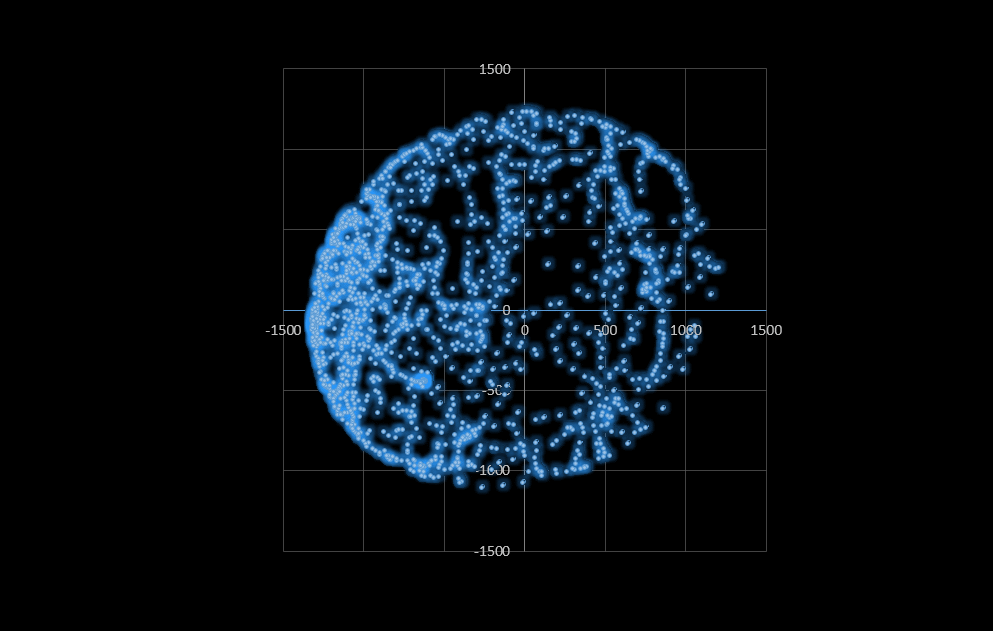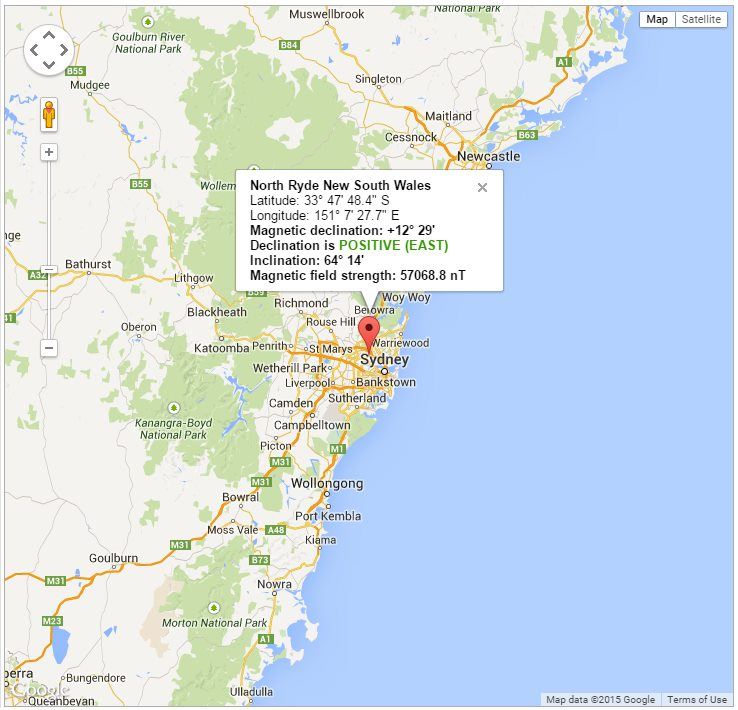# Create a Digital Compass with the Raspberry Pi – Part 3 – “Calibration”

Calibrating a magnetometer can improve the accuracy of the magnetometer readings.
All ferromagnetic materials will cause skew in the results of the measurements take by a magnetometer. This distortion falls into two categories, hard or soft iron distortion.

We can also further fine tune our readings by including the magnetic declination of our current location.

Git repository here
The code can be pulled down to your Raspberry Pi with;

pi@raspberrypi ~ \$ git clone https://github.com/ozzmaker/BerryIMU.git

The code for this guide can be found under the compass_tutorial03_calibration directory.# Hard Iron Distortion

Hard iron distortions are created by objects that produce a magnetic field. A speaker or piece of magnetized iron for example will cause a hard iron distortion. If the piece of magnetic material is physically attached to the same PCB as the magnetometer, then this type of hard iron distortion will cause a permanent bias in the sensor output.

## Soft Iron Distortion

Soft iron distortions are considered alterations in the existing magnetic field, this is usually caused by ferromagnetic materials around the sensor as well as the Earth's magnetic field (it is different in different locations).

Unfortunately, there is no easy way to calibrate for soft iron distortion and every time the sensor is moved to a new location, the calibration has to be done again.   Most people only apply hard iron calibration as this calibration usually stays constant.

An easy way to check to see if your sensor needs calibration is to plot the pairwise data from the raw X and Y readings.

The image below was created using Wolfram Mathematica on a Raspberry Pi. The data used was from an uncalibrated compass as.  Take note of the ellipsoid shape and how the center is not centered on the X,Y axis.

Ideally, our plot should be a  circle which is centered in the axis;

Hard iron distortion will be represent with the plot being off center;

Soft iron distortion will cause the plot to form an ellipse;

## Plotting your data with Wolfram Mathematica

We can use Wolfram Mathematical to plot our data, Mathematica is pre-installed with the Raspbian O/S.

We can use Wolfram Mathematical to plot our data, Mathematica is pre-installed with the Raspbian O/S.

1. Get your Raw data by using the code in our first compass tutorial, which can be found here.  You will want to pipe the output to a file, which we will use for Mathematica. Run the code with the command below for a few minutes. While it is running rotate the BerryIMU in all directions to try and gather as much information as you can.
pi@raspberrypi ~ \$ sudo ./compass_tutorial01 > ~/rawdatauncalibrated.txt
2. We now need to remove the first 10 and last line from the file as this may break the plotting. We can use this nifty awk 'one liner'.
pi@raspberrypi ~ \$ awk 'NR>10{ print l} {l=\$0}' ~/rawdatauncalibrated.txt > ~/uncalibrated.txt
3. You can now open Mathematica by clicking the icon on the desktop. Once it opens, click into the emty window and type the line in below.
```data = Import ["~/uncalibrated.txt", "Table", "FieldSeparators" -> {",", " "}];
ListPlot[data[[All, {6, 9}]], AspectRatio -> 1, Frame -> True]
```

Take note of the capital letters and the ';' at the end of the first line.

This is what is happening here;
-import our data, using "," and " "(Space) as field separators
-Do a pairwise plot
-Data[[All,{6,9}]] tells Mathematica to use all rows and columns 6 and 9 only.
-We want the output to have an aspect ratio of 1:1 and a border to be applied.

The above should be entered as two lines and at the end of the second line, hit 'shift-enter' to plot the data.  You can always go back and edit it and then press 'shift-enter' again.

If you have trouble using Mathematica, you can copy the data into Microsoft Excel and plot a scatter graph using the X and Y values.

## Calibration - Removing Hard Iron Distortion

Removing hard iron distortion is easy to do.  All that is required is to work out what the maximum and minimum readings from each axis is and then average them.   This new value then gets applied as an offset to the future readings taken from the magnetometer.

### Get your maximum and minimum values.

The Git repository linked above includes compass_tutorial03_calibration.c . This program can be used to work out what the maximum and minimum values are on your Magnetometer.

1. Compile the program ;
pi@raspberrypi ~ \$ gcc -o compass_tutorial03_calibration compass_tutorial03_calibration.c -lm
2. Run the program;
pi@raspberrypi ~ \$ sudo ./compass_tutorial03_calibration

While the program is running, the raw and maximum values will be printed to the screen. Keep rotating your magnetometer in random directions and until you see the values stop changing.

3. Stop the program with 'Ctrl-C' and you will see some definitions printed out in blue text which needs to be added to your compass program. E.g. compass_tutorial03.c in the above Git repository
```#define magXmax 1210
#define magYmax 1245
#define magZmax 1178
#define magXmin -1335
#define magYmin -1142
#define magZmin -1303```

### Apply hard iron offset in your code

Update your code to apply these offsets right after the raw readings are have been taken;

```                magRaw -= (magXmin + magXmax) /2 ;
magRaw -= (magYmin + magYmax) /2 ;
magRaw -= (magZmin + magZmax) /2 ;
```

## Calibration - Removing Soft Iron Distortion

Soft iron distortion is harder to remove and as stated before, can change depending what is around the magnetometer.  If your plot only has a small ellipsoid shape, I would not bother with applying the soft iron correction.

The code below is used to apply soft iron correction. The snippet of code also needs maximum and minimum values.

```                scaledMag  = (float)(magRaw - magXmin) / (magXmax - magXmin) * 2 - 1;
scaledMag  = (float)(magRaw - magYmin) / (magYmax - magYmin) * 2 - 1;
scaledMag  = (float)(magRaw - magZmin) / (magZmax - magZmin) * 2 - 1;
```

What this piece of code is doing is applying a scale to the raw readings based on an average.

```                float heading = 180 * atan2(scaledMag,scaledMag)/M_PI;
```

This plot shows a much better calibrated compass;

# Declination Correction

Go to this site http://magnetic-declination.com/ and enter in your location to find out what your magnetic declination is.

Mine is +12° 29' POSITIVEYou can then use Wolfram Alpha to get milliradians which we will use in our code

In the results on Wolfram Alpha look for the value express in mrad, mine is 217.9 mrad  (milliradians).
To apply this to your code;
1. Turn this value into Radians.

```                float declination = 217.9 / 1000.0;
```

2.Add the declination correction to our current heading. If you have a Negative declination, you need to minus it from your current heading.

```                heading += declination * 180/M_PI;
```

3.Correct the heading if declination forces it over 360.

```                if ( heading > 360)
```## 3 thoughts on “Create a Digital Compass with the Raspberry Pi – Part 3 – “Calibration””

1. M.Chavarín says:

Hi, your post is really understandable for us beginners. You made it really clear how to implement a hard and soft iron calibration, thanks for that.

2. Hi

data = Import ["~/calibrated.txt", "Table", "FieldSeparators" -> {",", " "}];
ListPlot[data[[All, {5,8}]]]

worked for me after editing raw data manually to remove extraneous leading and trailing lines in file.

File names in example will not work, since inputs and subsequent outputs are not matched 🙁

Awk input does not match output of generated file, and awk output does not match input of Wolfram input.

Thanks very much anyway!

This site uses Akismet to reduce spam. Learn how your comment data is processed.